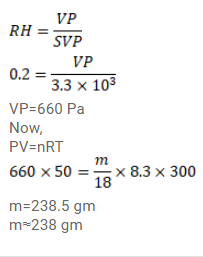# Use R=8.3 J/mol-K wherever required. The temperature and relative humidity in`
Question:

Use $\mathrm{R}=8.3 \mathrm{~J} / \mathrm{mol}-\mathrm{K}$ wherever required.

The temperature and relative humidity in a room are $300 \mathrm{~K}$ and $20 \%$ respectively. The volume of the room is $50 \mathrm{~m}^{3}$. The saturation vapour pressure at $300 \mathrm{~K}$ is $3.3 \mathrm{kPa}$. Calculate the mass of the water vapour present in the room.

Solution: